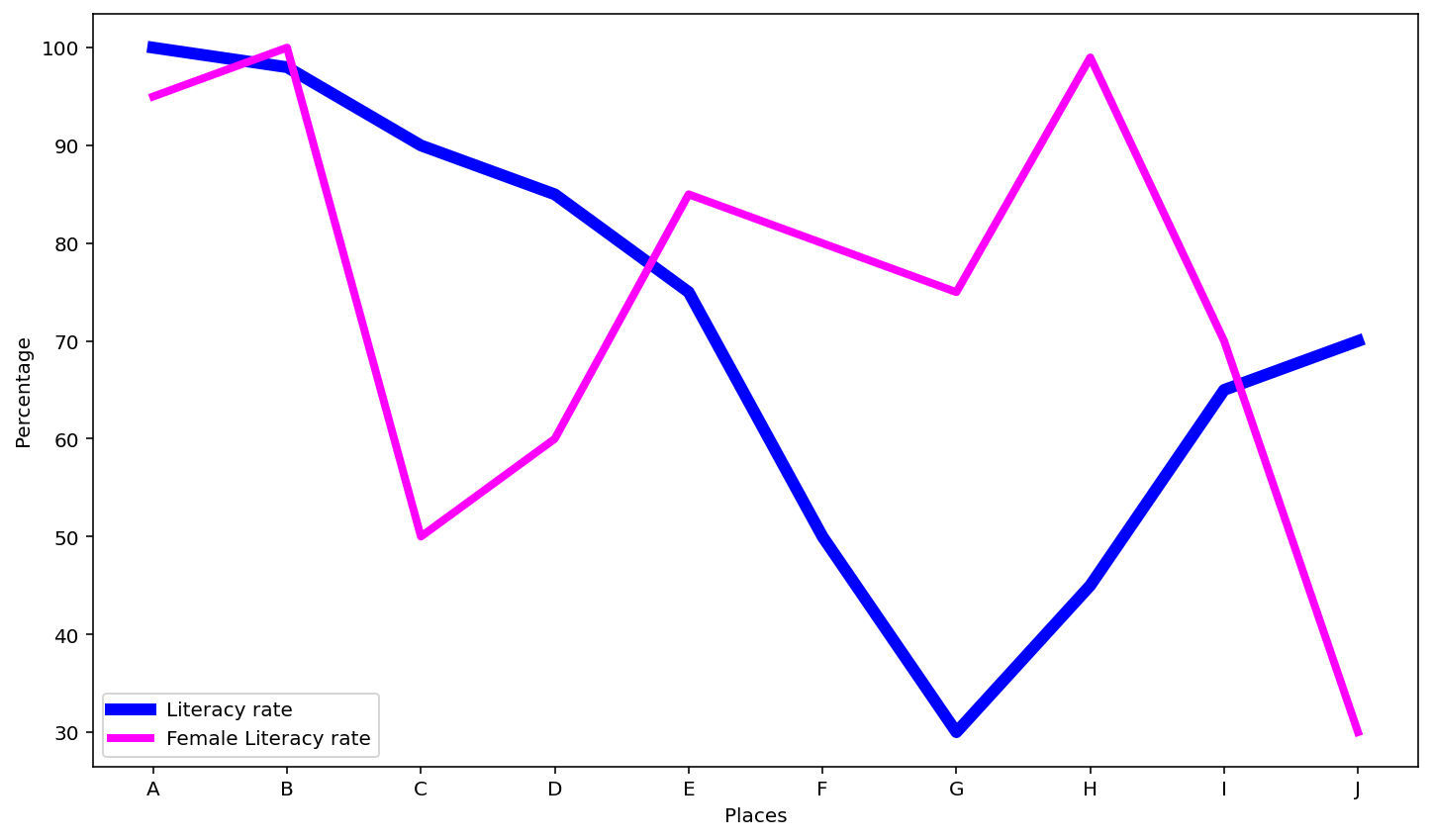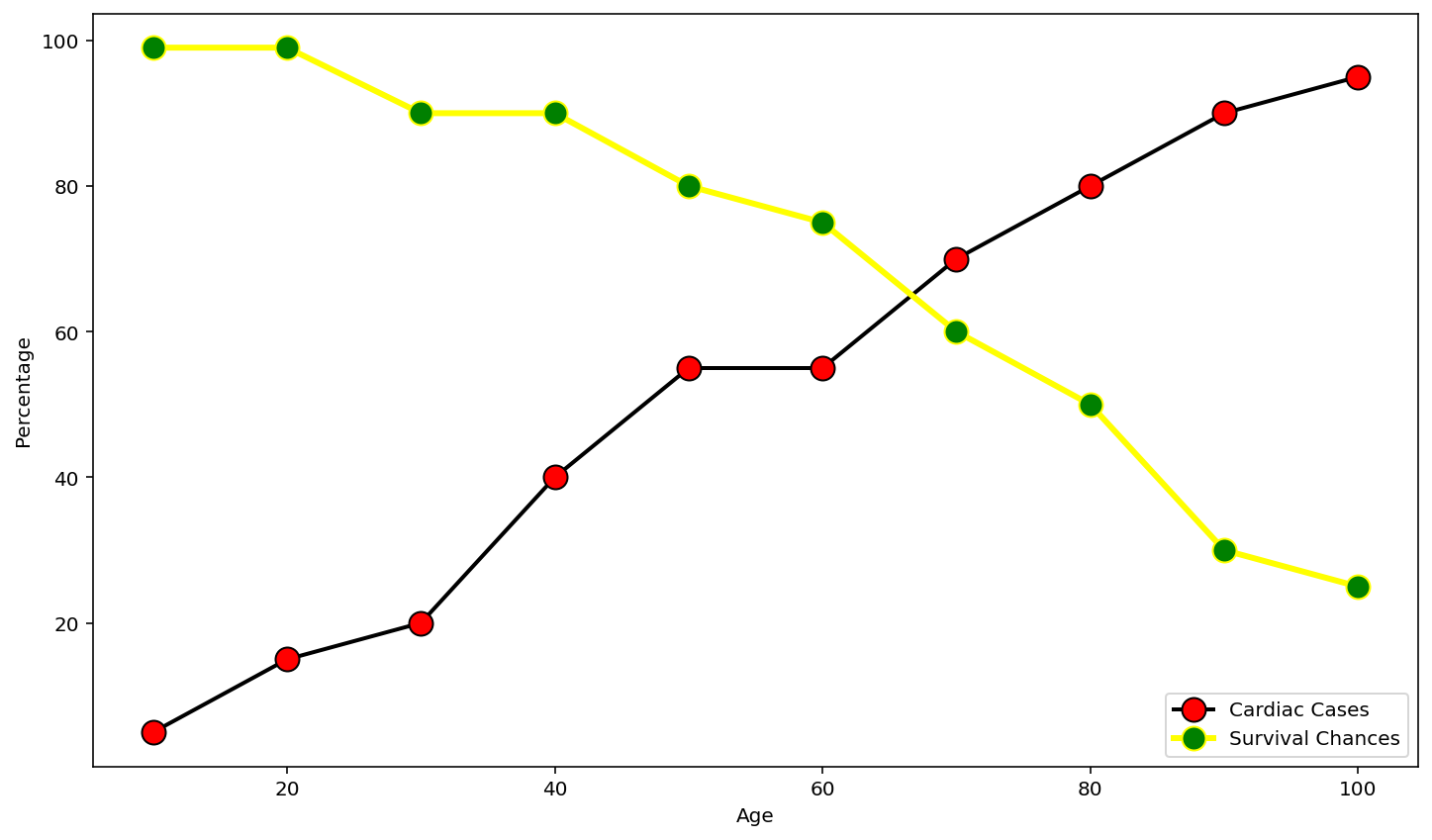# Increase the thickness of a line with Matplotlib

• Last Updated : 26 Dec, 2020

Prerequisites: Matplotlib

Matplotlib is the most widely used library for plotting graphs with the available dataset. Matplotlib supports line chart which are used to represent data over a continuous time span. In line chart, the data value is plotted as points and later connected by a line to show trend of a measure over time. The functionality of increasing the thickness of a line is given by linewidth attribute.

Linewidth: By default the linewidth is 1. For graphs with multiple lines it becomes difficult to trace the lines with lighter colors. This situation can be handled by increasing the linewidth. The linewidth can be used to focus on certain data compared to the others. It can help get a detailed visualization of the particular record in the dataset. This attribute belongs to plot function().

### Approach

• Import modules
• Create or load data
• Plot graph with required line thickness
• Display plot

### Functions used

• xlabel()- This function is used to set labels for x-axis

Syntax: plt.xlabel(xlabel, fontdict=None, labelpad=None, **kwargs)

Parameter:

• xlabel: accepts string type value and is used to label the X axis.
• fontdict: used to override default font properties of the label. Its default value is None and is optional.
• labelpad: default value is None. It is used to specify the spacing of the label from the axes. This is optional.
• **kwargs: used to specify other properties that can be used to modify the appearance of the label.
• ylabel()- this function is used to set labels for y-axis

Syntax: plt.ylabel(ylabel, fontdict=None, labelpad=None, **kwargs)

Parameter:

• ylabel: accepts string type value and is used to label the Y axis.
• fontdict: used to override default font properties of the label. Its default value is None and is optional.
• labelpad: default value is None. It is used to specify the spacing of the label from the axes. This is optional.
• **kwargs: used to specify other properties that can be used to modify the appearance of the label.
• plot()- It is used to make a 2D hexagonal binning plot of points x, y.

Syntax: plt.plot(x,y, data=None, **kwargs)

Parameter

• x,y : used to specify the data to be plotted along the x and y axis.
• data: default value is None. It is an object with labelled data and can be passed instead of the x,y values. If data object is passed then the xand y label should be specified.
• **kwargs: used to specify line properties like linewidth, color, antialiasing, marker, markercolor etc.
• legend()- A legend is an area describing the elements of the graph. In the matplotlib library, there’s a function called legend() which is used to Place a legend on the axes.

Syntax: plt.legend(**options)

Parameter

**options: used to specify the properties of the legend, its size, its location, edgecolor, facecolor etc

Example 1:

## Python3

 `import` `matplotlib.pyplot as plt`` ` `places ``=` `[``"A"``, ``"B"``, ``"C"``, ``"D"``, ``"E"``, ``"F"``, ``"G"``, ``"H"``, ``"I"``, ``"J"``]``literacy_rate ``=` `[``100``, ``98``, ``90``, ``85``, ``75``, ``50``, ``30``, ``45``, ``65``, ``70``]``female_literacy ``=` `[``95``, ``100``, ``50``, ``60``, ``85``, ``80``, ``75``, ``99``, ``70``, ``30``]`` ` `plt.xlabel(``"Places"``)``plt.ylabel(``"Percentage"``)`` ` `plt.plot(places, literacy_rate, color``=``'blue'``,``         ``linewidth``=``6``, label``=``"Literacy rate"``)`` ` `plt.plot(places, female_literacy, color``=``'fuchsia'``,``         ``linewidth``=``4``, label``=``"Female Literacy rate"``)`` ` `plt.legend(loc``=``'lower left'``, ncol``=``1``)`

OutputExample 2:

## Python3

 `import` `matplotlib.pyplot as plt`` ` `age ``=` `[``10``, ``20``, ``30``, ``40``, ``50``, ``60``, ``70``, ``80``, ``90``, ``100``]``cardiac_cases ``=` `[``5``, ``15``, ``20``, ``40``, ``55``, ``55``, ``70``, ``80``, ``90``, ``95``]``survival_chances ``=` `[``99``, ``99``, ``90``, ``90``, ``80``, ``75``, ``60``, ``50``, ``30``, ``25``]`` ` `plt.xlabel(``"Age"``)``plt.ylabel(``"Percentage"``)`` ` `plt.plot(age, cardiac_cases, color``=``'black'``, linewidth``=``2``,``         ``label``=``"Cardiac Cases"``, marker``=``'o'``, markerfacecolor``=``'red'``, markersize``=``12``)`` ` `plt.plot(age, survival_chances, color``=``'yellow'``, linewidth``=``3``,``         ``label``=``"Survival Chances"``, marker``=``'o'``, markerfacecolor``=``'green'``, markersize``=``12``)`` ` `plt.legend(loc``=``'lower right'``, ncol``=``1``)`

OutputMy Personal Notes arrow_drop_up